# How to Calculate and Solve for Einsten Relative Apparent Viscosity (for Dilute System or Suspension) | Rheology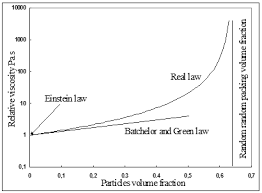The image above represents einstein relative apparent viscosity.

To compute for einstein relative apparent viscosity, one essential parameter is needed and these parameter is Solid Landing (φ).

The formula for calculating einstein relative apparent viscosity:

ηra = 1 + 25φ

Where:

ηra = Einstein Relative Apparent Viscosity
φ = Solid Landing

Let’s solve an example;
Find the einstein relative apparent viscosity when the solid landing is 24.

This implies that;

φ = Solid Landing = 24

ηra = 1 + 25φ
ηra = 1 + 25 x 24
ηra = 1 + 600
ηra = 601

Therefore, the einstein relative apparent viscosity is 601.

Calculating for Solid Landing when the Einstein Relative Apparent Viscosity is Given.

φ = ηra – 1 / 25

Where;

φ = Solid Landing
ηra = Einstein Relative Apparent Viscosity

Let’s solve an example;
Given that the einstein relative apparent viscosity is 56. Find the Solid landing?

This implies that;

ηra = Einstein Relative Apparent Viscosity = 56

φ = ηra – 1 / 25
φ = 56 – 1 / 25
φ = 55 / 25
φ = 2.2

Therefore, the solid landing is 2.2.

Nickzom Calculator – The Calculator Encyclopedia is capable of calculating the einstein relative apparent viscosity.

To get the answer and workings of the einstein relative apparent viscosity using the Nickzom Calculator – The Calculator Encyclopedia. First, you need to obtain the app.

You can get this app via any of these means:

To get access to the professional version via web, you need to register and subscribe for NGN 2,000 per annum to have utter access to all functionalities.
You can also try the demo version via https://www.nickzom.org/calculator

Apple (Paid) – https://itunes.apple.com/us/app/nickzom-calculator/id1331162702?mt=8
Once, you have obtained the calculator encyclopedia app, proceed to the Calculator Map, then click on Materials and Metallurgical under Engineering.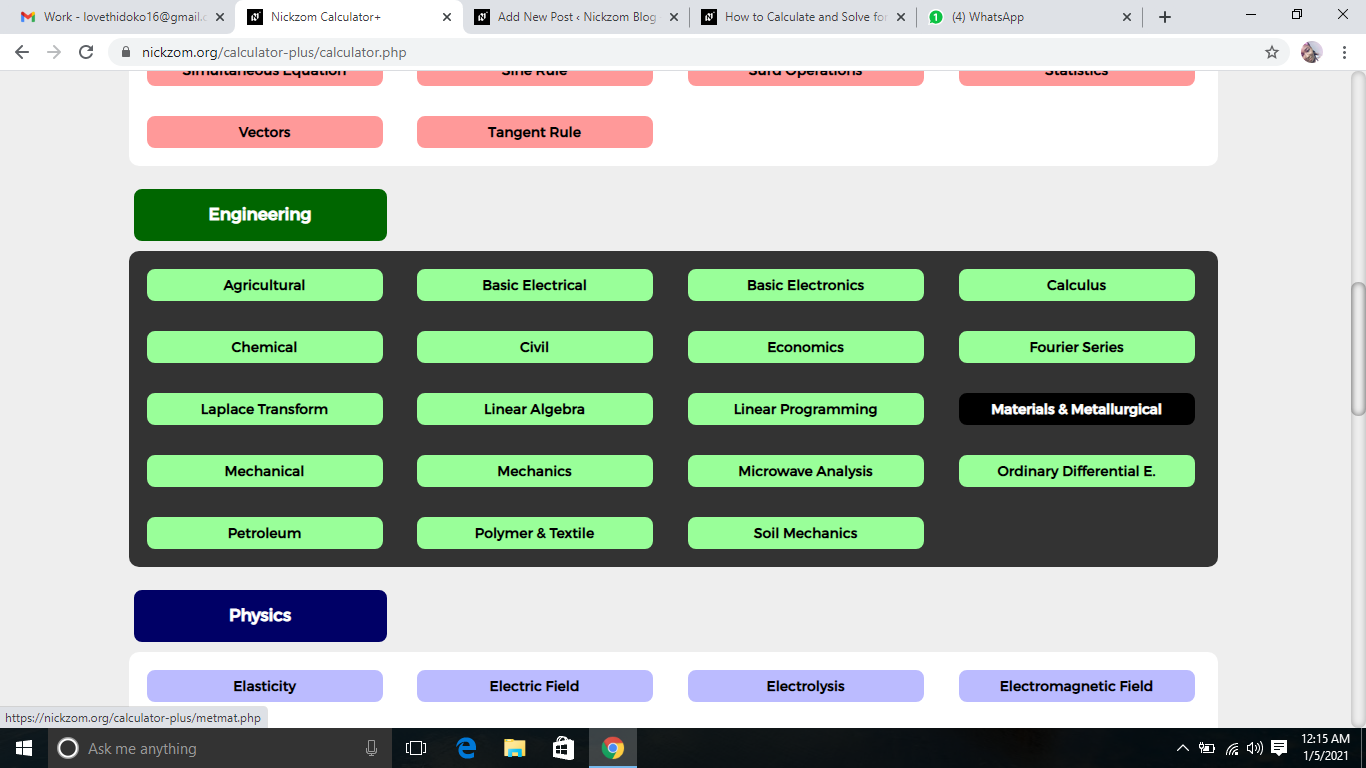Now, Click on Rheology under Materials and Metallurgical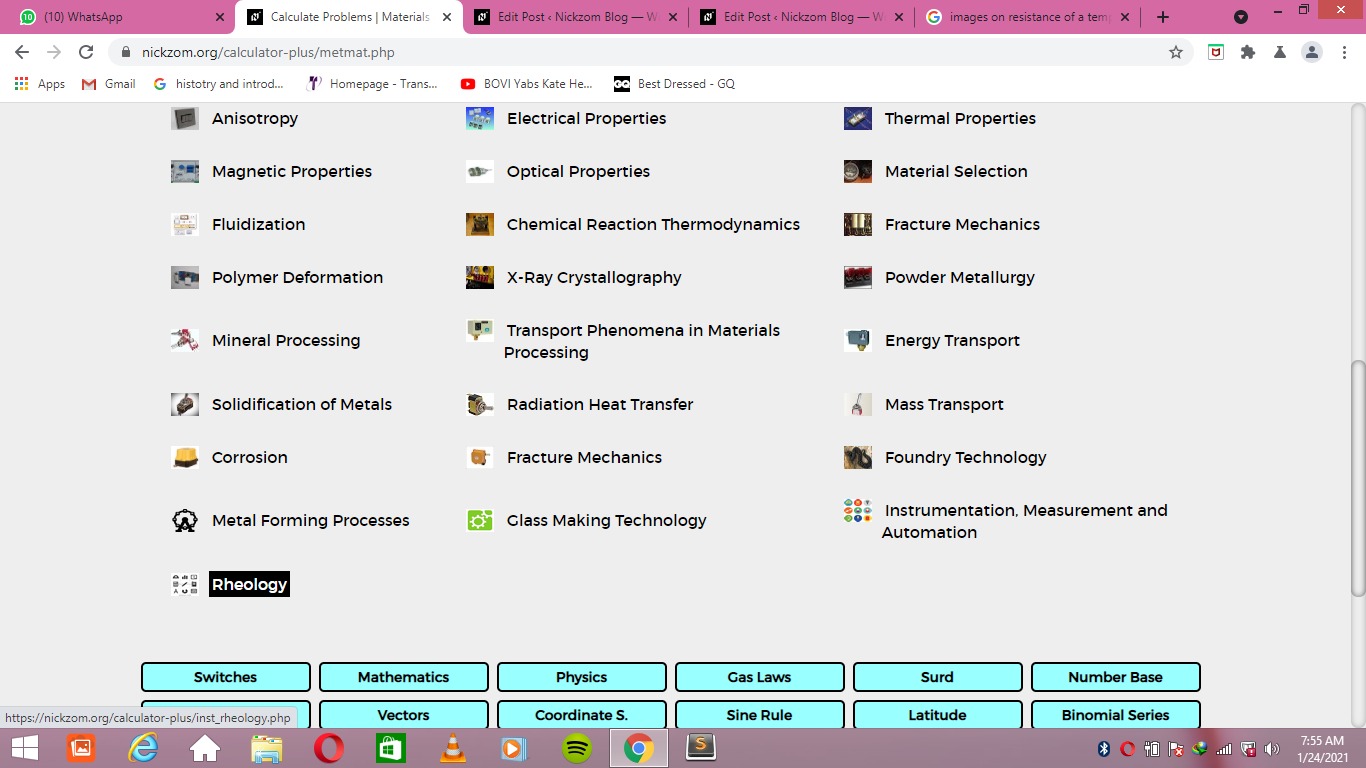Now, Click on Einstein Relative Apparent Viscosity under RheologyThe screenshot below displays the page or activity to enter your value, to get the answer for the einstein relative apparent viscosity according to the respective parameter which is the Solid Landing (φ).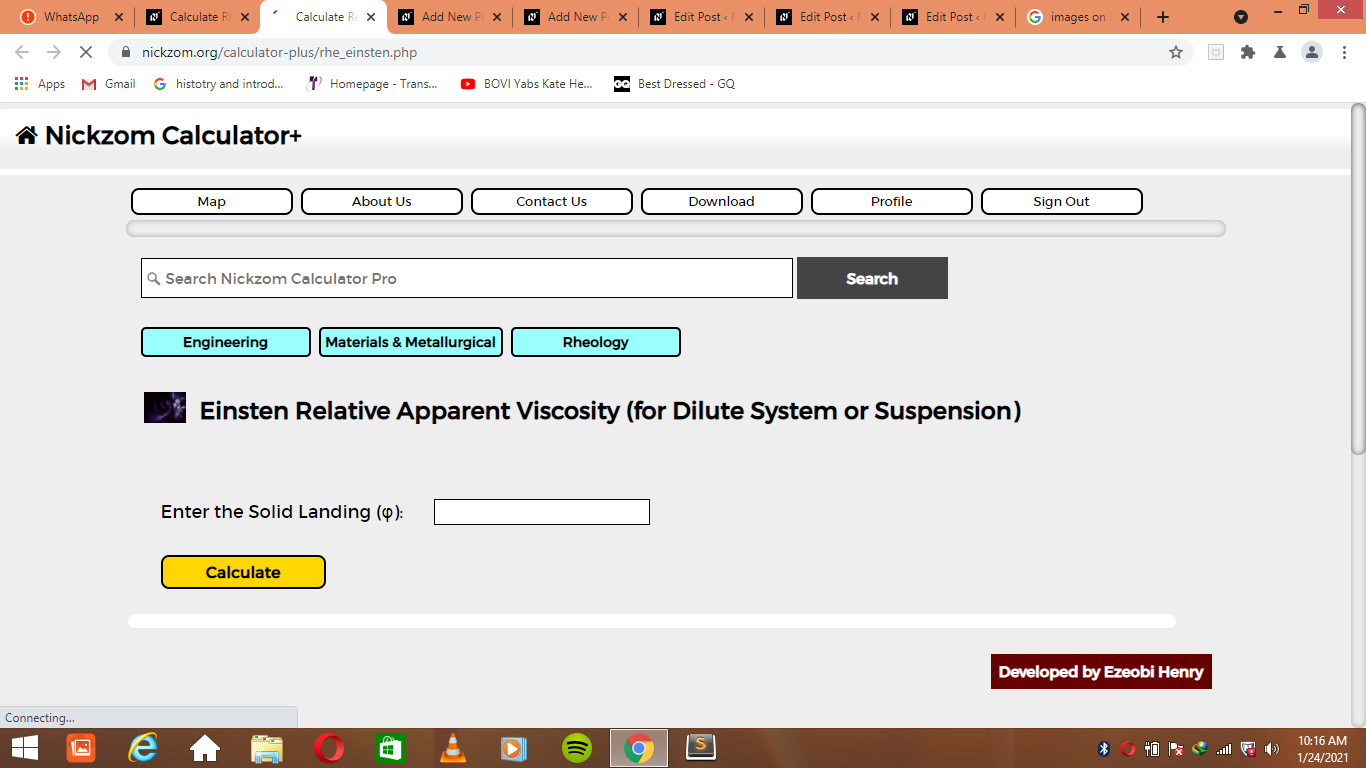Now, enter the value appropriately and accordingly for the parameter as required by the Solid Landing (φ) is 24.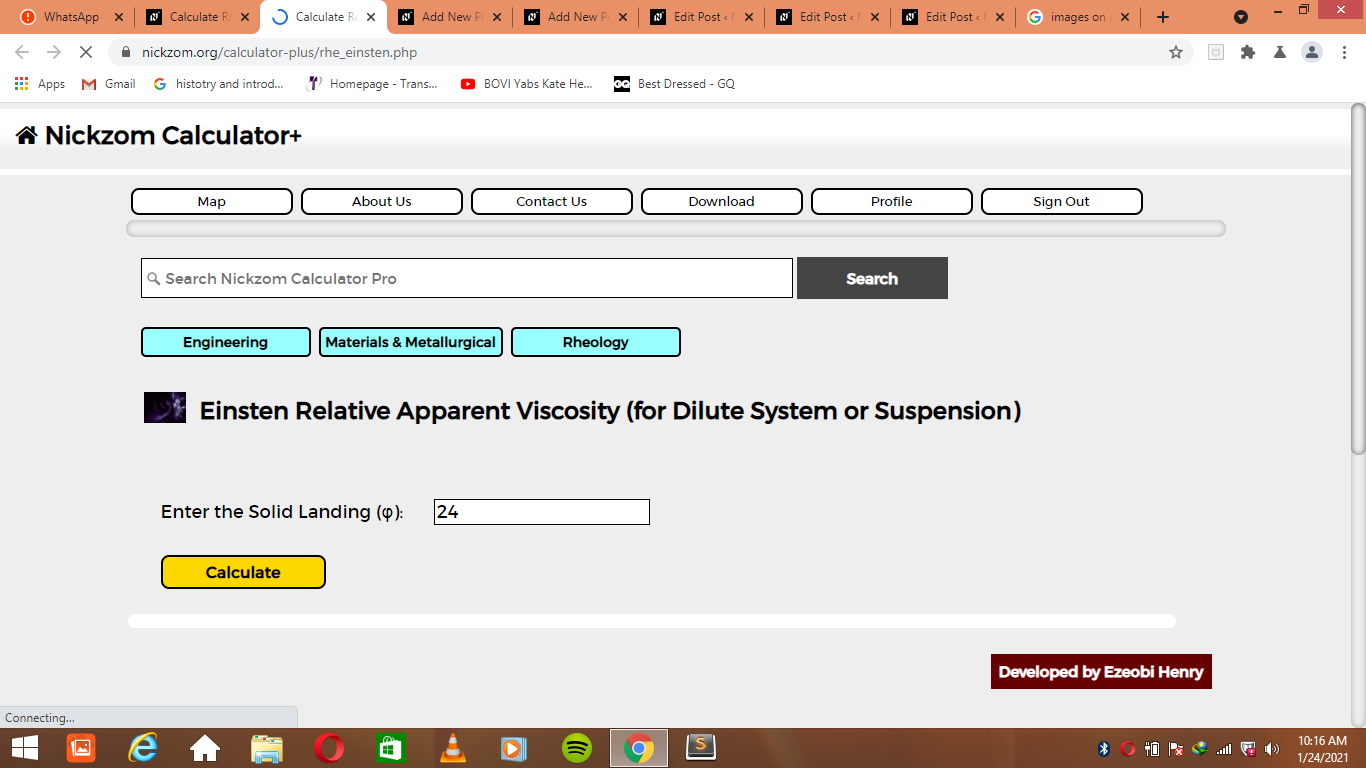Finally, Click on Calculate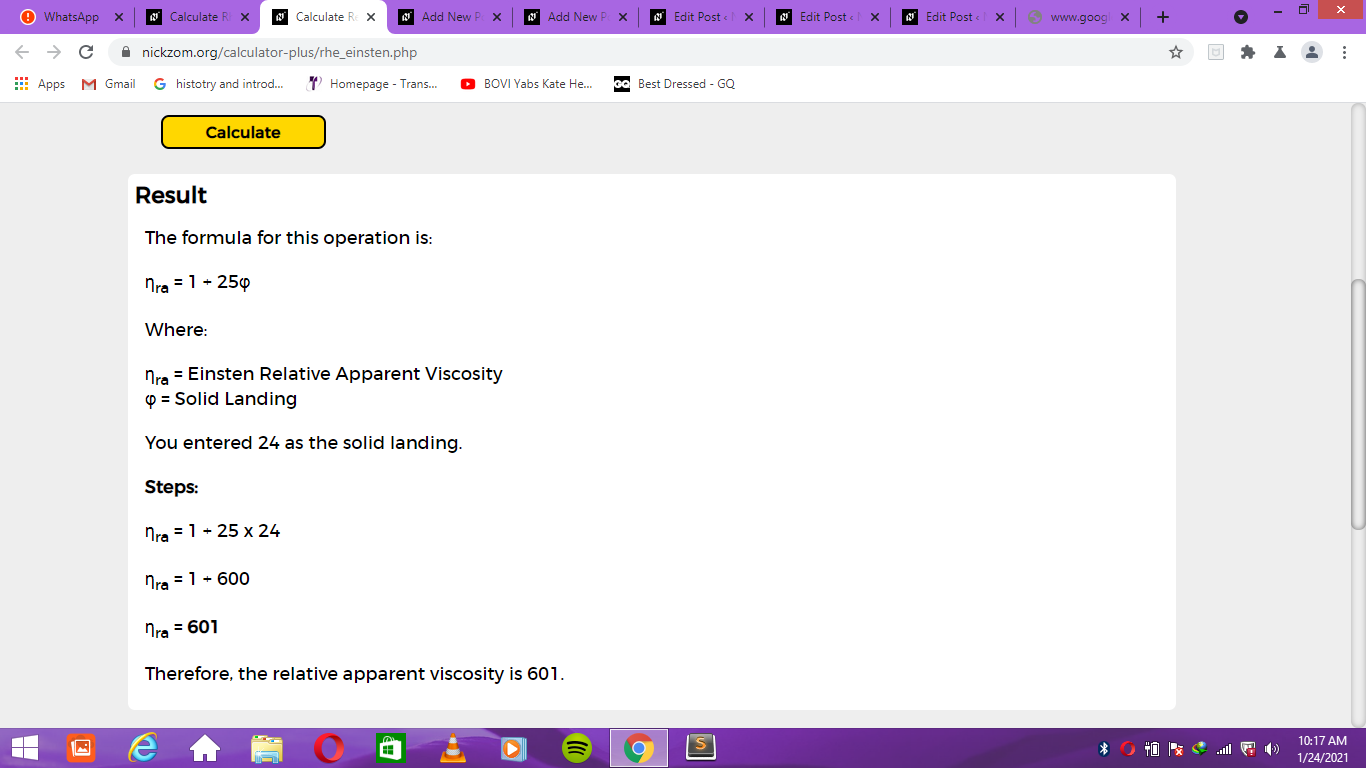As you can see from the screenshot above, Nickzom Calculator– The Calculator Encyclopedia solves for the einstein relative apparent viscosity and presents the formula, workings and steps too.